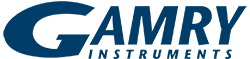# Square-wave Voltammetry

## Introduction

Among the many electrochemical techniques available in Gamry Instruments’ Framework™ software is square-wave voltammetry, a form of pulse voltammetry. This Application Note describes what square-wave voltammetry is, and the parameters involved.

### Theory Behind Square-wave Voltammetry

We start with a staircase voltage series applied to a sample. Added to the staircase waveform is a square wave, so that as the voltage suddenly jumps with each step, the square wave is coincident with the jump. Halfway through the step, the square wave reverses polarity. This repeated series of staircase-plus-square-wave creates a characteristic voltage sequence applied to the sample as shown in Fig. 1.

Cell current is measured over time between the counter and working electrodes. Cell voltage is measured between the working and reference electrodes.Fig. 1. Schematic of how voltage varies with time in square-wave voltammetry.

Time, τ, for One Square-Wave Cycle and Step

Both square-wave cycle and length of a single step in the voltage series take a time τ. The inverse of the cycle length is the frequency, 1/τ.

Scan Rate

The scan rate for such an experiment is inversely dependent upon the time per step, τ:During the scan, the current is recorded at the end of the forward pulse and at the end of the reverse pulse, meaning it is sampled twice per cycle. Waiting till the end of the pulse to sample the current avoids involving the charging current.

The frequency, f, used in square-wave voltammetric experiments is generally from about 1 to 125 Hz. Such a high f means that square-wave voltammetry is usually much faster than other pulsed experiments.

Experiment setup

Below is an example of the experimental setup window* from Gamry Instruments’ Framework™ software:Fig. 2. Experiment setup window for square-wave voltammetry in Gamry Instruments’ Framework software.
Sampling Mode (circled in red) has three choices, including Surface mode.

Surface Mode

In a digital sweep the standard convention is to acquire one data point at the very end of each step. Gamry Instruments calls this method Fast mode. Such a method of sampling discriminates against any capacitive current or surface-bound reactions. The current caused by any capacitive charging or faradaic current confined to the surface decays in the initial part of the step and does not contribute to the measured current.

A second acquisition method is Noise Reject, averages over the last 20% of each step. When compared to Fast mode, this improves the signal-to-noise ratio while still capturing current primarily from diffusing species.

With Surface mode, Gamry Instruments enables a unique sampling method to eliminate the differences between a staircase and a true ramp. In Surface mode, the data are sampled throughout the duration of the step, and then averaged. This allows capturing both capacitive effects and any faradaic effects confined to the surface. (See our Application Note “Measuring Surface Related Currents using Digital Staircase Voltammetry.”) No other potentiostat manufacturer includes a Surface mode capability.

For square-wave voltammetric measurements involving surface reactions, we recommend using Surface mode during scanning.

Plotting

The software subtracts the two current measurements, and plots this difference (Idiff) against the applied staircase potential (Vstep). The result is that this technique gives peaks caused by faradaic processes. The height of the peak is directly related to the solution concentration of that species.

#### Examples

We provide an example of a trace of Cd+2 (6 ppm) dissolved in aqueous acetate buffer solution. We ran a square-wave voltammetry experiment at τ = 0.1 s (i.e., frequency = 10 Hz) on a Gamry Instruments potentiostat. The experiment required only 6.1 s (61 points at 0.1 s each). Data are presented as Idiff (the difference between forward and reverse currents) versus Vstep in Fig. 3.Fig. 3. Square-wave voltammetry at τ = 0.1 s for 6 ppm Cd+2 in an aqueous acetate buffer.

Were we to vary the concentration of Cd+2, the peak height would be proportional to [Cd+2]. Adding another ion in solution (e.g., Pb+2) would add a second peak at a different Vstep.

Quantitative Measurements of Trace Copper

In this example, various concentrations of copper ions in the parts-per-million range dissolved in acidified aqueous solution were scanned by square-wave voltammetry, along with a blank. This series of experiments (see Table 1) shows how the concentration of trace amounts of copper is directly related to the peak height.Fig. 4. Various concentrations of acidified copper ions in solution, recorded with square-wave voltammetry. Gray is the blank.

Table 1. Concentrations of copper and their respective peak heights from square-wave voltammetry. R2 = 0.9755.

#### Peak height (µA)at –250 mV

Scan 1 88 0.408
Scan 2  25  0.7536
Scan 3  50 1.205
Scan 4  77  1.432
Scan 5 100   1.738

Comparison of Three Acquisition Modes

To show the extra sensitivity in Surface mode exclusive to Gamry Instruments’ potentiostats, Figure 5 presents a background-subtracted set of scans using a biosensor recorded in all three modes: Fast, Noise Reject, and Surface.Fig. 5. Square-wave voltammetry at 100 Hz recorded in three acquisition modes on a biosensor.
Blue is Fast mode, Red is Noise Reject mode, and Purple is Surface mode. All data are background-subtracted.

Clearly Surface mode is far and away the method to see the best signal for surface-related reactions.

### Conclusion

Square-wave voltammetry is a rapid means of qualitatively and quantitatively determining analyte even at low concentrations in a solution.